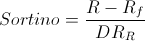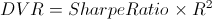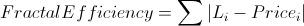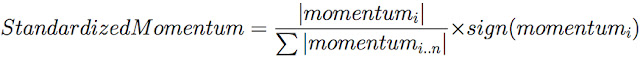Select Page

# Dynamic Asset Allocation for Practitioners, Part 3: Risk-Adjusted Momentum

[latexpage]
Risk is in the Eye of the Beholder

So far, we’ve discussed the importance of investment universe selection and price momentum in designing a robust asset allocation methodology. If you haven’t read those articles, we would strongly encourage you to do so before proceeding with this one. We lay most of the explanatory and theoretical groundwork for this article in the previous instalments, and we won’t repeat them here.

In our previous article, we examined how we can use total return momentum to identify asset classes with a higher probability of delivering positive performance in the near future. As a result, by regularly rotating into the strongest performing asset classes, we demonstrated that investors can earn a substantial return premium, with more consistent results and smaller drawdowns, than more conventional portfolios.  The article looked at multiple ways of measuring an asset’s trajectory, but with limited regard for the path it took.

In this article, we examine whether it pays to account for differences in the path assets take to produce their momentum. All other things equal, do investors express a short-term preference for assets that have produced their returns with less risk, where risk is measured broadly as having delivered a smoother ride?  As you will see, there are a variety of ways to quantify the investor’s experience, including different measures of both return and risk. We wish to explore the question of whether investors show risk aversion in manifesting the momentum effect, and which type of risk they most seek to avoid.

As a reminder, to safeguard ourselves form the temptations of “curve fitting,” we will run every risk-adjusted momentum method through a battery of simulations that vary the number of assets in the universe, portfolio concentration, trading frequency, and trading day.  In total, each method will be tested on 16,116 simulation variations.  The best methodologies will have to show promise across a broad set of these tests. Readers should be cautioned not to pay attention to small differences in performance across methodologies, which are almost certainly due to randomness over the test horizon. Rather, we should seek to determine if investors pay attention to risk at all when expressing the behaviors that manifest in the momentum effect, and whether any one type of risk stands out.

## Defining Our Momentum Metrics

We will present 13 different risk-adjusted momentum measures selected from among the most popular and widely-available methodologies, both parametric and non-parametric.

As a refresher, parametric analysis assumes the data follows a specific probability distribution (think bell curve). Conversely, non-parametric analysis makes no initial assumptions about the distribution of returns. The true distribution of market returns is an area of intense debate, so is helpful to present both methodologies for comparison.

The following is a breakdown of each of the momentum indicators tested. To avoid any confusion, we use the same notation for each. Below are some recurring elements:

• n – The “lookback” parameter. Simply the number of trading days we are observing to make our measurement.
• t – The most current price, return, or period.
• R – The set of returns in a given lookback period.
• \$overline{R}\$ The mean (average) return in a given lookback.
• \$R_f\$ The “risk-free” return as measured by the T-bill yield.

Metric 1: Sharpe Ratio

Created by William Sharpe, this ratio is perhaps the most widely-used method of risk adjustment. It measures the asset returns minus the risk-free returns (i.e. excess returns), divided by the standard deviation of returns.Metric 2: Omega Ratio

The Omega Ratio separates return observations into those that are above and below a certain threshold. It then divides the cumulative returns above the threshold by the cumulative returns below.Metric 3: Sortino Ratio

A modified version of the Sharpe ratio. While the Sharpe ratio considers all volatility of an asset’s returns, the Sortino ratio penalizes only the volatility of downside returns. This involves dividing excess return by downside semi-deviation, to arrive at the final measurement.Metric 4: Calmar Ratio (MAR)

Originally published by the Managed Accounts Reports the Calmar ratio divides annualized return by maximum drawdown observed over the lookback period.Metric 5: David Varadi Ratio (DVR)

Here we are concerned not only with the lookback’s risk-adjusted returns, but the linearity of the return trajectory. It is computed by multiplying the Sharpe ratio by the coefficient of determination, of the cumulative log returns.Metric 6: Value at Risk (VaR)

Used widely in the financial industry for measuring firm-wide risk and exposure, Value at Risk calculates, for a given confidence level a, the magnitude of expected loss. If we sort our lookback returns from least to greatest, the Value At Risk is the return observation at the a percentile. Note that this method measures the so-called empirical VaR, which is distinct from parametric VaR, which is more common and assumes a certain return distribution.Metric 7: Conditional Value at Risk (CVaR)

A variation of the VaR, the Conditional Value at Risk is simply the mean value of all return observations at the lower left tail of the return distributions, between the maximum loss and a given confidence level a. Theoretically, it does a better job of capturing the true tail risk.Metric 8: Max Loss Ratio

Given the set of returns from the lookback, we divide the mean return by minimum return as a measure of risk.Metric 9: Average Drawdown Ratio

Similar to the Max Loss Ratio, but instead of dividing by the lookback’s smallest return, we use the average drawdown.Metric 10: High Low Differential

This novel risk-adjusted momentum metric captures the current position relative to the highest and lowest prices observed over the lookback horizon.

Metric 11: Ulcer Price Index (UPI)

Created by Peter Martin in 1987, this risk measure takes into account both the magnitude of losses and the time spent in drawdown. The following is the pseudocode for computing the Ulcer Index (UI).

SumSq = 0
MaxValue = 0
for T = 1 to NumOfPeriods do
if Value[T] > MaxValue then MaxValue = Value[T]
else SumSq = SumSq + sqr(100 * ((Value[T] / MaxValue) – 1))
UI = sqrt(SumSq / NumOfPeriods)

Metric 12: Gain to Pain Ratio

Popularized by the book Hedge Fund Market Wizards by Jack Schwager, this ratio takes the sum of all positive returns and divides it by the sum of all the negative returns in the lookback.Metric 13: Fractal Efficiency

Created by Perry Kaufman, this ratio expresses the view that trend strength is more related to linearity than trajectory.  It is calculated as the lookback’s n-day net change in price divided by the sum of the absolute values of the n-day net change in price. In practice, it is strongly related to the Sharpe ratio.As in our previous post, we will aggregate the momentum scores across asset classes independently at each lookback. We will then calculate each asset’s contribution to the total aggregate momentum measured across all assets, by dividing each instrument’s score by the sum of the absolute values of all the scores in the group. These scores are then averaged across each lookback to arrive at a final score for each asset.[latexpage]
Analysis of Performance Distributions

The following table describes the median result for each strategy, simulated from 1991 through March 2017, on daily total return data across all simulation variations.

Figure 1: Median Performance by Momentum MethodologySource: Global Financial Data, CSI Data, ReSolve Asset Management

Perhaps surprisingly, there doesn’t seem to be a material difference in performance between any of the thirteen methods. They all work about equally well, judging by median performance. Of course, the median is only one way to gauge performance. Let’s examine the total range of outcomes from all 209,508 tests. The following charts show the simulation results for each strategy at each decile of terminal wealth. Pay special attention to the visual dispersion of results across the deciles, as this provides a potential clue about the stability of the method.

Figures 2-15: Decile Equity Lines of Momentum MethodsSource: Global Financial Data, CSI Data, ReSolve Asset Management

In contrast with the performances of price momentum, our risk-adjusted methods display much smaller dispersion, in general. Visually, Sharpe, average drawdown (avgdd), and high-low-differential (highlowdiff) methods seem to have produced the tightest distribution of results, though most of the methods appear relatively stable.

The following charts are cumulative quantile plots of the performance statistics across all combinations of each momentum system. In our opinion, the most realistic way to evaluate the performance of a system is at the most punitive end of the distribution. In other words, we want to focus on methods that perform the best when they are at their worst. As traditional tests for statistical significance focus on the 5th percentile of outcomes, we have highlighted the strategies with the best results at that level.

Figures 16-28. Compound Annual Growth Rate by QuantileSource: Global Financial Data, CSI Data, ReSolve Asset Management

Figures 29 – 41. Sharpe Ratio by QuantileSource: Global Financial Data, CSI Data, ReSolve Asset Management

Figures 42 – 54. Maximum Drawdown by QuantileSource: Global Financial Data, CSI Data, ReSolve Asset Management

It is also useful to observe the distribution of results for different levels of portfolio concentration, and different rebalance frequencies/holding periods. The following charts break down the average compound returns (CAGR), Sharpe ratios, and maximum drawdowns across all the momentum methods, along each of these dimensions. Of particular note, whereas price momentum showed a clear difference based on portfolio concentration, we see almost no difference in results between portfolios that hold top 2, 3 or 4 assets at each rebalance. Likewise, the rebalancing periods have little to no overall effect, though we do see small a drop-off in Sharpe ratio for monthly rebalancing.

Figures 55 – 57. Average CAGR, Sharpe, and Max Drawdown Across Holding ConcentrationsSource: Global Financial Data, CSI Data, ReSolve Asset Management

Figures 58 – 60. Average CAGR, Sharpe, and Max Drawdown Across Rebalancing PeriodsSource: Global Financial Data, CSI Data, ReSolve Asset Management

[latexpage]
Indicator Diversification

We know from observing the distribution of performance statistics reported thus far that, while the different risk-adjusted momentum signals produce similar results on average, they deliver peaks and troughs at slightly different times. But just how different are these indicators, statistically speaking? In Figure 61 we averaged the daily returns of all 16,116 combinations of each strategy to construct a correlation matrix.

Figure 61. Pairwise Correlations among Momentum MethodologiesSource: Global Financial Data, CSI Data, ReSolve Asset Management

The correlations run from a minimum of 0.79 (between the High Low Differential and the Average Drawdown), to a high of .99 between several different methods (note: these methods are mathematically almost identical). The average pairwise correlation across strategies is 0.945.

It’s hard to imagine that a system composed of such highly-correlated strategies could offer an edge over any of the individual strategies. Despite this intuition, an aggregate index provides a material boost to risk-adjusted performance relative to even the best individual strategy. As Figure 62 shows, this hypothetical meta-momentum strategy delivers the highest Sharpe ratio, and lowest volatility and max drawdown, while offering very similar compound returns.  It even represents an improvement in risk-adjusted performance relative to the meta price-momentum strategy from article 2, which sported a Sharpe of 1.23.

Even within a single factor, diversification works.

Figure 62. Aggregate Performance of all 209,508 Simulation Variations.Source: Global Financial Data, CSI Data, ReSolve Asset Management

## Risk-Adjusted Momentum is a Useful Indicator.  So What Comes Next?

In order to isolate the momentum factor, up to now we have only focused on holding assets in equal weight at each lookback.  In our upcoming posts, we abandon this restriction and introduce a variety of algorithms to vary our position sizing.  In doing so, we hope to further enhance the performance of each method and provide an additional layer of insight on how to construct an effective Dynamic Asset Allocation strategy.

We will incorporate heuristic as well as more complex optimization procedures to identify optimal sizing combinations, offering the practitioner a variety of ways to achieve their – or their clients’ – financial goals.

Stay tuned.